## 哈弗 哈弗H1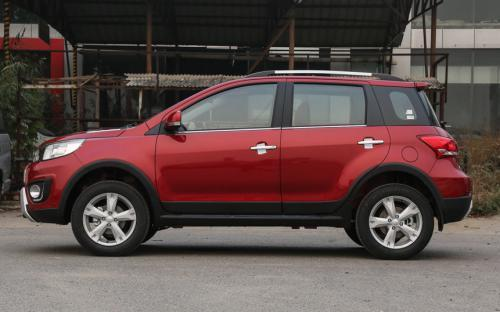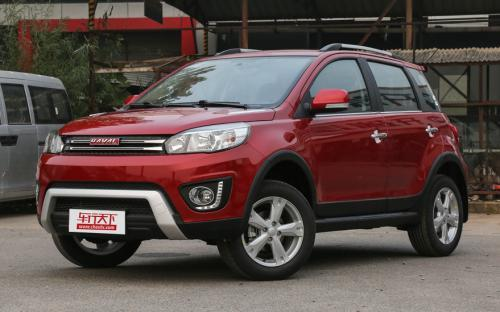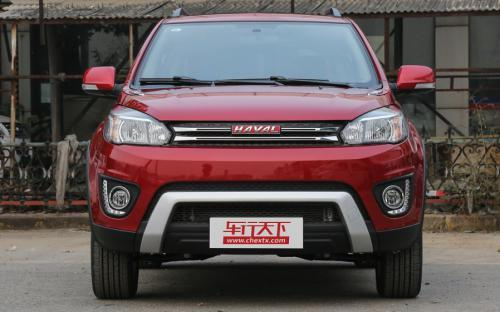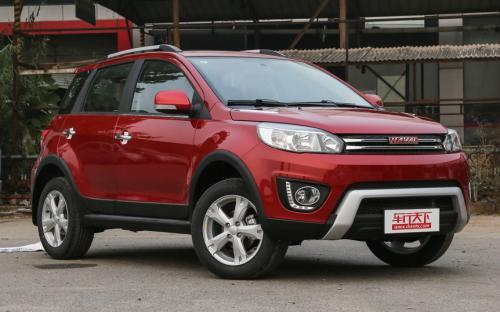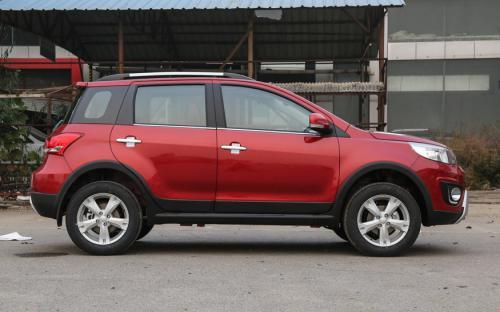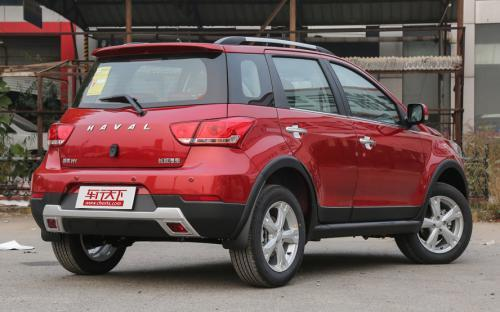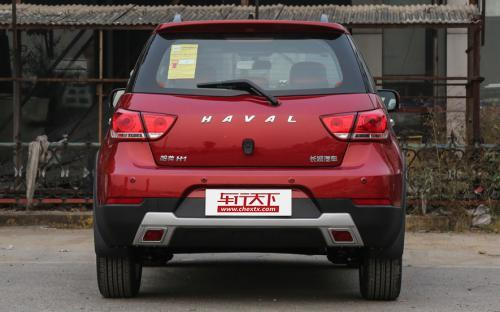0 种颜色可选2017款最低售价：5.49 万元起

3995(mm)1728(mm)1617(mm)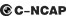##### 配置亮点：
• 胎压监测装置

• ISOFIX儿童座椅接口

• 车身稳定控制(ESC/ESP/DSC等)

• 电动天窗

• 定速巡航

• 后倒车雷达

• 真皮座椅

• GPS导航系统

• 氙气大灯

• 后视镜加热

2017款 红标 1.5L AMT舒适型 (130张)哈弗 哈弗H1 在售车型

排量 车型 厂商指导价 本地最低报价 购车工具
1.5L 红标 1.5L 手动标准型 5挡手动 5.49万 5.49万

询底价+对比
红标 1.5L 手动舒适型 5挡手动 5.99万 5.99万

询底价+对比
红标 1.5L 手动豪华型 5挡手动 6.39万 询底价+对比
红标 1.5L AMT舒适型 6挡AMT 6.29万 询底价+对比
红标 1.5L AMT豪华型 6挡AMT 6.79万 6.79万

询底价+对比

哈弗 哈弗H1 经销商

查看更多 >>
更多 >>

本地降价排名

### 哈弗 哈弗H1 动力加速

哈弗H1 0-100公里加速时间分布在 0.0-秒 属于 超跑级

动力级别 加速时间 车型

哈弗 哈弗H1 视频

哈弗 哈弗H1 新闻资讯

# 2016款蓝标哈弗H1上市 售6.49-7.89万

国产新车 超过7263次关注

长城汽车官方宣布，2016款蓝标哈弗H1正式上市，新车在外观细节方面有所调整，推出了搭载1.5L发动机的共计8款车型，售价区间为6.49-7.89万元。

# 哈弗H1首月突破6000台

新闻中心 超过3939次关注

11月销量出炉，长城汽车销量再爆多重亮点。哈弗品牌销售5.4万辆，增长达98%，相比去年同期几乎翻番。11月初上市的哈弗H1和哈弗H9再成SUV瞩目焦点——哈弗H1上市首月...

# 一起看看“年轻化”的小型SUV哈弗H1

新闻中心 超过4706次关注

哈弗H2大家已不再陌生，它的百搭恰好迎合了年轻人追求潮流、个性的心理，7月销1260、8月销3859、9月销8288，10月成功突破1.1万辆，进入SUV市场前五。

# 配置抢眼 SUV佼佼者哈弗H1性能解析

行业动态 超过5389次关注

继今年7月份哈弗H2推出之后，就在11月3日，哈弗家族最小的成员哈弗H1如约而至，呈现在众人眼前。作为国内自主SUV品牌的佼佼者，哈弗品牌推出的这款小型SUV在配置上...

# 300台哈弗H1开启抢购 被抢购一空

行业动态 超过5464次关注

2014年11月11日，300台哈弗H1在哈弗商城开启抢购，不到两天就被狂热的消费者一扫而空。

猜你喜欢

﻿
• 快速找车
• 选择品牌
• 选择品牌
• A  奥迪
• A  阿斯顿·马丁
• A  阿尔法·罗密欧
• B  宝沃
• B  布加迪
• B  巴博斯
• B  保时捷
• B  宾利
• B  奔驰
• B  宝马
• B  本田
• B  别克
• B  标致
• B  比亚迪
• B  宝骏
• B  北汽制造
• B  北汽新能源
• B  北汽幻速
• B  北汽威旺
• B  北京汽车
• B  奔腾
• B  北汽绅宝
• C  长安
• C  长安商用
• C  长城
• C  昌河
• D  大众
• D  道奇
• D  DS
• D  东南
• D  东风风神
• D  东风风行
• D  东风小康
• D  东风风度
• D  东风
• F  福特
• F  丰田
• F  菲亚特
• F  法拉利
• F  福田
• F  福迪
• F  福汽启腾
• G  观致
• G  广汽传祺
• G  广汽吉奥
• G  GMC
• H  红旗
• H  汉腾汽车
• H  哈弗
• H  哈飞
• H  海格
• H  海马
• H  华颂
• H  黄海
• H  华泰
• H  恒天
• J  吉利汽车
• J  捷豹
• J  Jeep
• J  江淮
• J  江铃
• J  金杯
• J  九龙
• J  金旅
• K  凯翼
• K  凯迪拉克
• K  克莱斯勒
• K  科尼塞克
• K  卡威
• K  开瑞
• L  路虎
• L  林肯
• L  劳斯莱斯
• L  兰博基尼
• L  雷克萨斯
• L  铃木
• L  雷诺
• L  理念
• L  力帆
• L  莲花汽车
• L  猎豹
• L  路特斯
• L  陆风
• M  马自达
• M  MG
• M  MINI
• M  玛莎拉蒂
• M  摩根
• M  迈凯轮
• N  纳智捷
• O  欧宝
• O  讴歌
• O  欧朗
• Q  奇瑞
• Q  起亚
• Q  启辰
• R  日产
• R  荣威
• R  瑞麒
• S  三菱
• S  斯威汽车
• S  萨博
• S  smart
• S  斯柯达
• S  斯巴鲁
• S  思铭
• S  双龙
• S  上汽大通
• S  双环
• T  特斯拉
• T  腾势
• W  沃尔沃
• W  五菱汽车
• W  五十铃
• W  威兹曼
• W  威麟
• X  现代
• X  雪佛兰
• X  雪铁龙
• X  西雅特
• Y  一汽
• Y  英菲尼迪
• Y  英致
• Y  依维柯
• Y  野马汽车
• Y  永源
• Z  众泰
• Z  中华
• Z  中兴
• Z  知豆
• 选择车系
• 选择车系
• 车型对比
• 选择品牌
• 选择品牌
• A  奥迪
• A  阿斯顿·马丁
• A  阿尔法·罗密欧
• B  宝沃
• B  布加迪
• B  巴博斯
• B  保时捷
• B  宾利
• B  奔驰
• B  宝马
• B  本田
• B  别克
• B  标致
• B  比亚迪
• B  宝骏
• B  北汽制造
• B  北汽新能源
• B  北汽幻速
• B  北汽威旺
• B  北京汽车
• B  奔腾
• B  北汽绅宝
• C  长安
• C  长安商用
• C  长城
• C  昌河
• D  大众
• D  道奇
• D  DS
• D  东南
• D  东风风神
• D  东风风行
• D  东风小康
• D  东风风度
• D  东风
• F  福特
• F  丰田
• F  菲亚特
• F  法拉利
• F  福田
• F  福迪
• F  福汽启腾
• G  观致
• G  广汽传祺
• G  广汽吉奥
• G  GMC
• H  红旗
• H  汉腾汽车
• H  哈弗
• H  哈飞
• H  海格
• H  海马
• H  华颂
• H  黄海
• H  华泰
• H  恒天
• J  吉利汽车
• J  捷豹
• J  Jeep
• J  江淮
• J  江铃
• J  金杯
• J  九龙
• J  金旅
• K  凯翼
• K  凯迪拉克
• K  克莱斯勒
• K  科尼塞克
• K  卡威
• K  开瑞
• L  路虎
• L  林肯
• L  劳斯莱斯
• L  兰博基尼
• L  雷克萨斯
• L  铃木
• L  雷诺
• L  理念
• L  力帆
• L  莲花汽车
• L  猎豹
• L  路特斯
• L  陆风
• M  马自达
• M  MG
• M  MINI
• M  玛莎拉蒂
• M  摩根
• M  迈凯轮
• N  纳智捷
• O  欧宝
• O  讴歌
• O  欧朗
• Q  奇瑞
• Q  起亚
• Q  启辰
• R  日产
• R  荣威
• R  瑞麒
• S  三菱
• S  斯威汽车
• S  萨博
• S  smart
• S  斯柯达
• S  斯巴鲁
• S  思铭
• S  双龙
• S  上汽大通
• S  双环
• T  特斯拉
• T  腾势
• W  沃尔沃
• W  五菱汽车
• W  五十铃
• W  威兹曼
• W  威麟
• X  现代
• X  雪佛兰
• X  雪铁龙
• X  西雅特
• Y  一汽
• Y  英菲尼迪
• Y  英致
• Y  依维柯
• Y  野马汽车
• Y  永源
• Z  众泰
• Z  中华
• Z  中兴
• Z  知豆
• 选择车系
• 选择车系
• 选择车型
• 选择车型
• 意见反馈